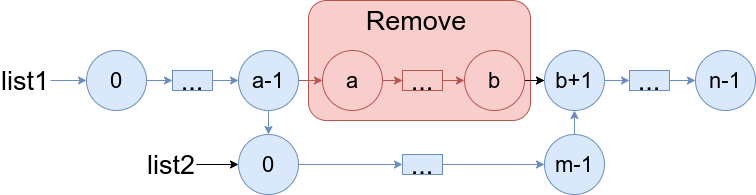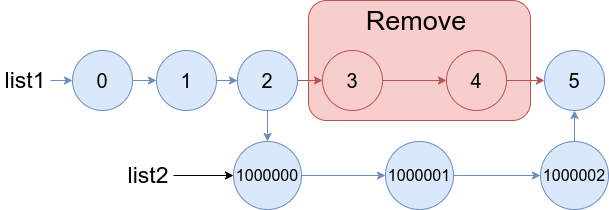# 1669. Merge In Between Linked Lists

Medium
You are given two linked lists: `list1` and `list2` of sizes `n` and `m` respectively.
Remove `list1`'s nodes from the `ath` node to the `bth` node, and put `list2` in their place.
The blue edges and nodes in the following figure indicate the result:Build the result list and return its head.
Example 1:Input: list1 = [0,1,2,3,4,5], a = 3, b = 4, list2 = [1000000,1000001,1000002]
Output: [0,1,2,1000000,1000001,1000002,5]
Explanation: We remove the nodes 3 and 4 and put the entire list2 in their place. The blue edges and nodes in the above figure indicate the result.
Example 2:Input: list1 = [0,1,2,3,4,5,6], a = 2, b = 5, list2 = [1000000,1000001,1000002,1000003,1000004]
Output: [0,1,1000000,1000001,1000002,1000003,1000004,6]
Explanation: The blue edges and nodes in the above figure indicate the result.
Constraints:
• `3 <= list1.length <= 10^4`
• `1 <= a <= b < list1.length - 1`
• `1 <= list2.length <= 10^4`

### 解題

func mergeInBetween(list1 *ListNode, a int, b int, list2 *ListNode) *ListNode {
cur1, cur2 := list1, list2
a1, b1 := list1, list1
index := 0
for cur1 != nil{
if index == a - 1{
a1 = cur1
} else if index == b {
b1 = cur1.Next
break
}
cur1 = cur1.Next
index++
}
a1.Next = cur2
for cur2 != nil{
if cur2.Next == nil{
cur2.Next = b1
break
}
cur2 = cur2.Next
}
return list1
}